## 1 Faster R-CNN 简介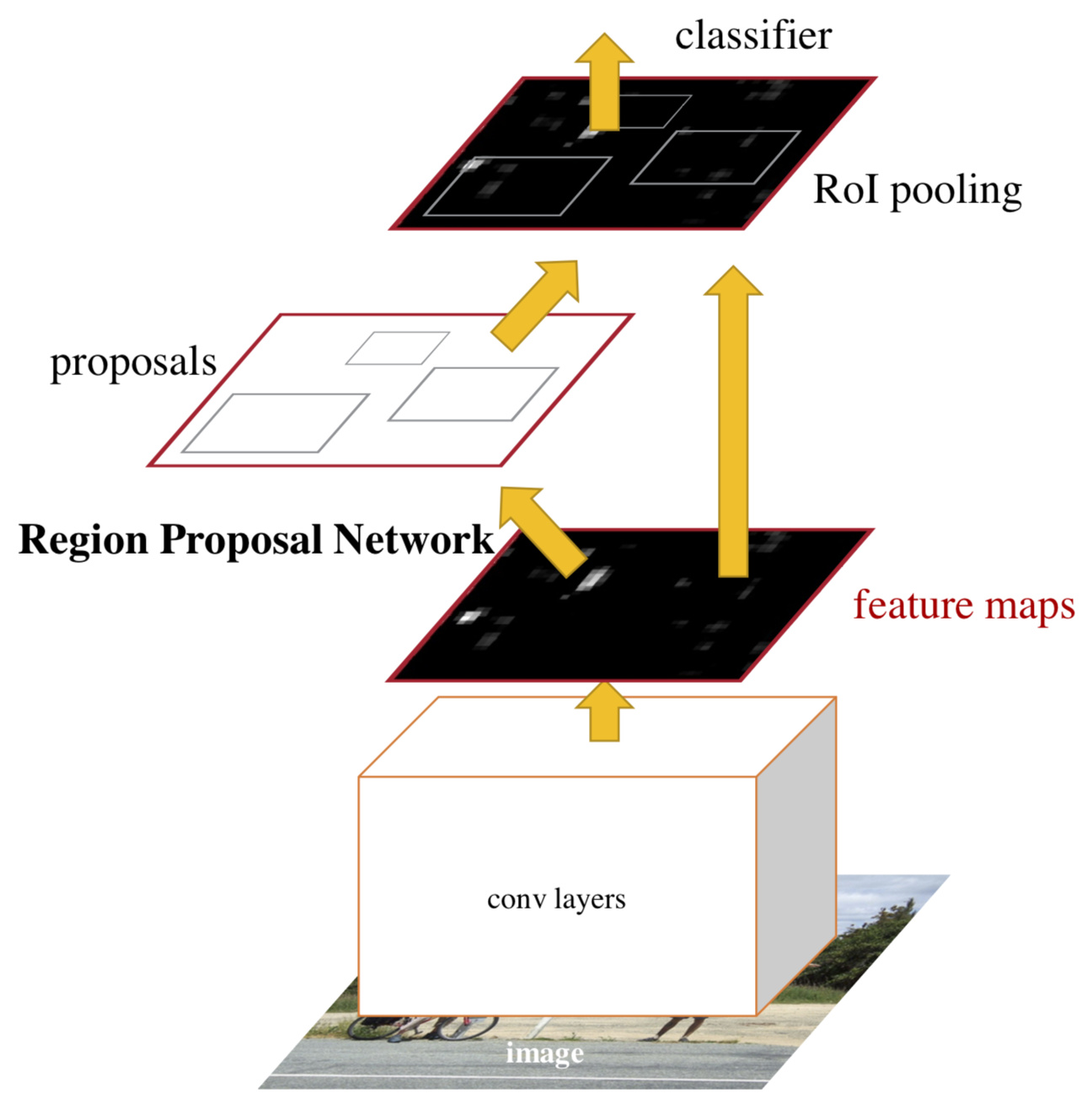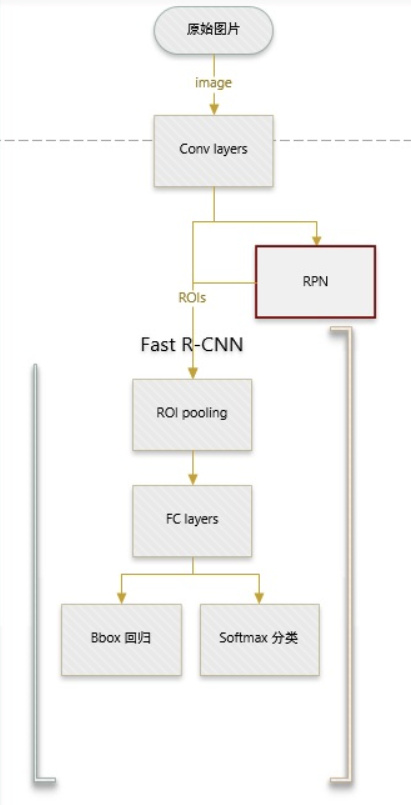Faster RCNN 可以分为4个主要内容：

• Conv layers。作为一种CNN网络目标检测方法，Faster RCNN首先使用一组基础的conv+relu+pooling层提取image的feature maps。该feature maps被共享用于后续RPN层和全连接层。
• Region Proposal Networks。RPN网络用于生成region proposals。该层通过softmax判断anchors属于foreground或者background，再利用bounding box regression修正anchors获得精确的proposals。
• Roi Pooling。该层收集输入的feature maps和proposals，综合这些信息后提取proposal feature maps，送入后续全连接层判定目标类别。
• Classification。利用proposal feature maps计算proposal的类别，同时再次bounding box regression获得检测框最终的精确位置。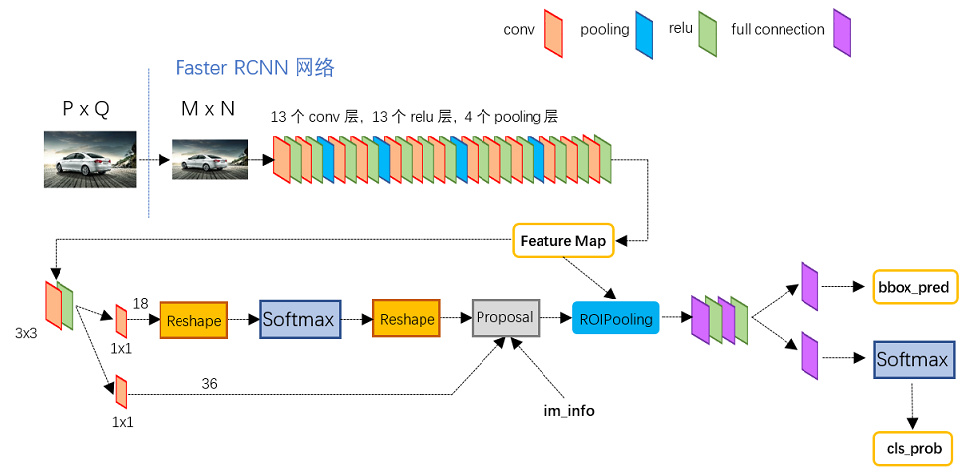RPN网络首先经过 $$3\times 3$$ 卷积，再分别生成foreground anchors与bounding box regression偏移量，然后计算出proposals；

## 2 Conv layers

Conv layers包含了conv，pooling，relu三种层。以python版本中的VGG16模型中的faster_rcnn_test.pt的网络结构为例，如图2，Conv layers部分共有13个conv层，13个relu层，4个pooling层。这里有一个非常容易被忽略但是又无比重要的信息，在Conv layers中：

1. 所有的conv层都是：kernel_size=3，pad=1
2. 所有的pooling层都是：kernel_size=2，stride=2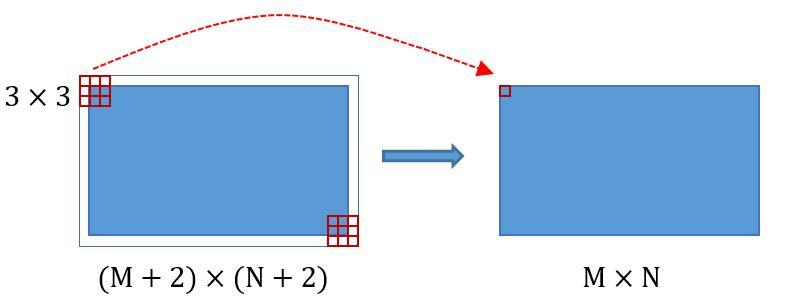## 3 Region Proposal Networks (RPN)

RCNN解决的是，“为什么不用CNN做classification呢？”
Fast R-CNN解决的是，“为什么不一起输出bounding box和label呢？”
Faster R-CNN解决的是，“为什么还要用selective search呢？”

RPN的核心思想是使用CNN卷积神经网络直接产生Region Proposal，使用的方法本质上就是滑动窗口（只需在最后的卷积层上滑动一遍）。

RPN的输入是任意大小的图像，输出是一组打过分的候选框（object proposals）。在卷积的最后一层feature map上使用固定大小的窗口（注意sliding window只是选择位置，除此之外没有其它作用，和后面的3*3的卷积区分）滑动，每个窗口会输出固定大小维度的特征（图中256)，每一个窗口对候选的9个box进行回归坐标和分类（这里的分类表示box中是不是一个object，而不是具体的类别）。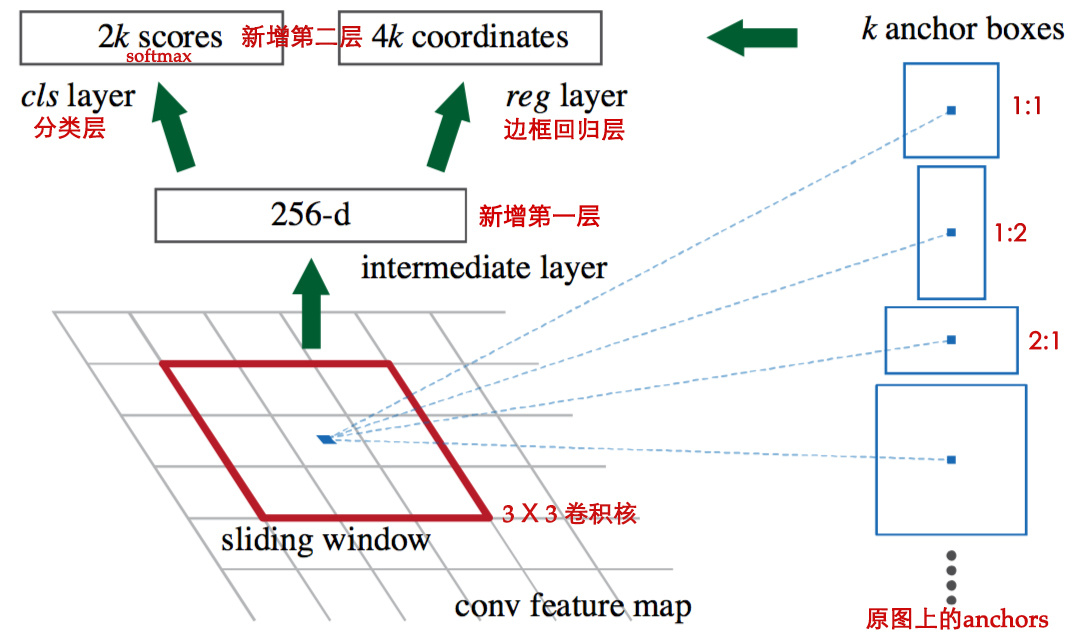1. 上面一条通过softmax分类anchors获得foreground和background（检测目标是foreground）
2. 下面一条用于计算对于anchors的bounding box regression偏移量，以获得精确的proposal。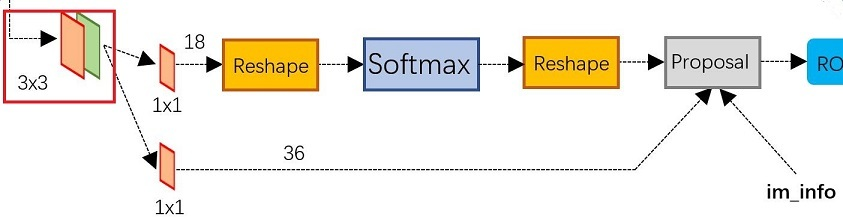### 3.1 anchors

Anchors是一组大小固定的参考窗口：
$$三种尺度 (128^2，256^2，512^2)\times 三种长宽比(1:1，1:2，2:1)$$ ，如下图所示，表示RPN网络中对特征图滑窗时每个滑窗位置所对应的原图区域中9种可能的大小，相当于模板，对任意图像任意滑窗位置都是这9中模板。继而根据图像大小计算滑窗中心点对应原图区域的中心点，通过中心点和size就可以得到滑窗位置和原图位置的映射关系，由此原图位置并根据与Ground Truth重复率贴上正负标签，让RPN学习该Anchors是否有物体即可1. 在原文中使用的是ZF model中，其Conv Layers中最后的conv5层num_output=256，对应生成256张特征图，所以相当于feature map每个点都是256-d
2. 在conv5之后，做了RPN_conv/3x3卷积且num_output=256，相当于每个点又融合了周围3x3的空间信息，同时256-d不变（如第四张图中的红框）
3. 假设在conv5 feature map中每个点上有 $$k$$ 个anchor（默认k=9），而每个anhcor要分foreground和background，所以每个点由256d feature转化为 $$cls=2k$$ scores；而每个anchor都有 $$[x, y, w, h]$$ 对应4个偏移量，所以 $$reg=4k$$ coordinates
4. 补充一点，全部anchors拿去训练太多了，训练程序会选取256个合适的anchors进行训练（什么是合适的anchors下文有解释）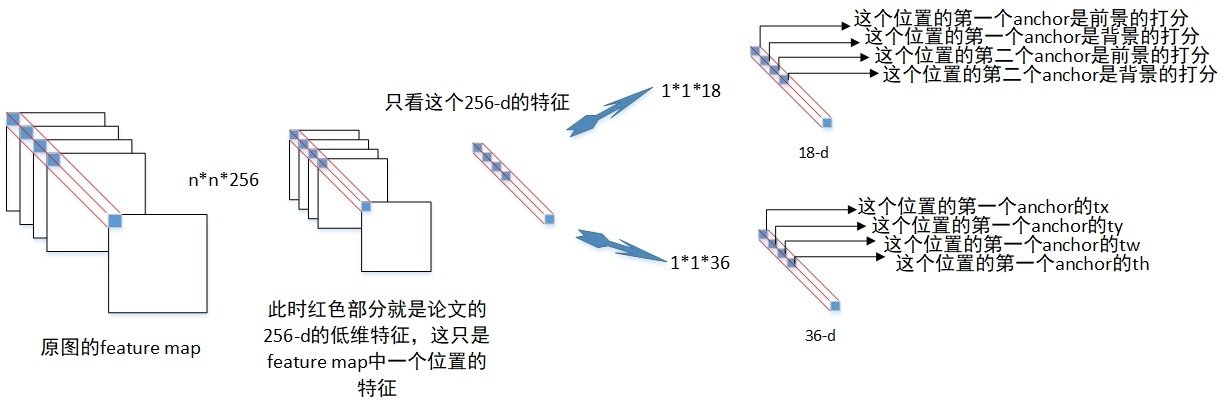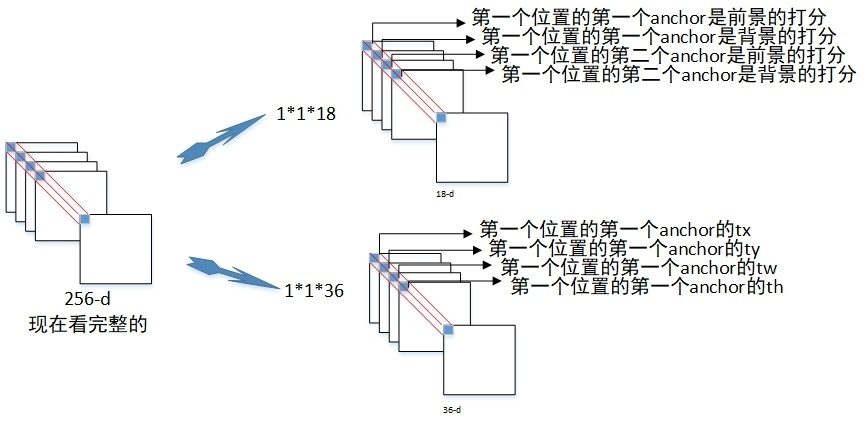1. 对每个标定的ground true box区域，与其重叠比例最大的anchor记为 正样本 (保证每个ground true 至少对应一个正样本anchor)
2. 对1剩余的anchor，如果其与某个标定区域重叠比例大于0.7，记为正样本（每个ground true box可能会对应多个正样本anchor。但每个正样本anchor 只可能对应一个grand true box）；如果其与任意一个标定的重叠比例都小于0.3，记为负样本。
3. 对1,2剩余的anchor，弃去不用。
4. 跨越图像边界的anchor弃去不用

### 3.2 bounding box regression 原理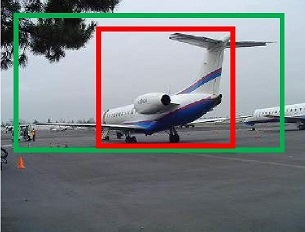$$f(A_x, A_y, A_w, A_h)=(G’x, G’y, G’w, G’h)≈(Gx, Gy, Gw, Gh)$$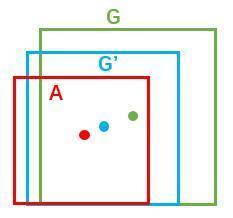1. 先做平移 $$(\Delta x, \Delta y)$$，$$\Delta x = A_w d_x(A), \Delta y = A_h d_y(A)$$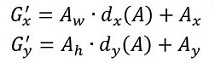1. 再做缩放 $$(S_w, S_h)$$，$$S_w = exp(d_w(A)), S_h = exp(d_h(A))$$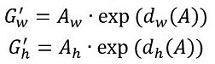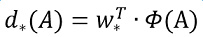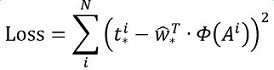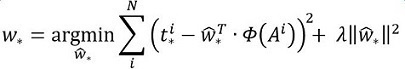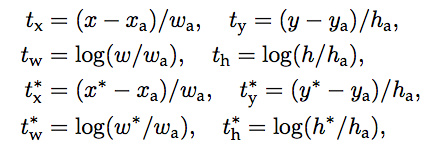$$x,y,w,h\ 为\ box\ 的中心坐标，宽，高$$

$$\begin{cases} x: predicted\ box\ (就是Proposal)\\ x_{a}: anchor\ box\ (N\times M \times k 个)\\ x^{*}: Ground\ Truth\ (正确标定的GT)\ \end{cases}$$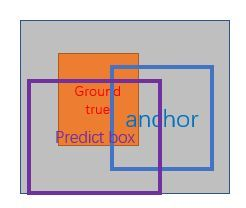### 3.3 Proposal Layer

Proposal Layer负责综合所有 $$[d_{x}(A)，d_{y}(A)，d_{w}(A)，d_{h}(A)]$$ 变换量和foreground anchors，计算出精准的proposal，送入后续RoI Pooling Layer。

Proposal Layer有3个输入：fg/bg anchors分类器结果rpn_cls_prob_reshape，对应的bbox reg的[dx(A)，dy(A)，dw(A)，dh(A)]变换量rpn_bbox_pred，以及im_info；另外还有参数feat_stride=16，这和图4是对应的。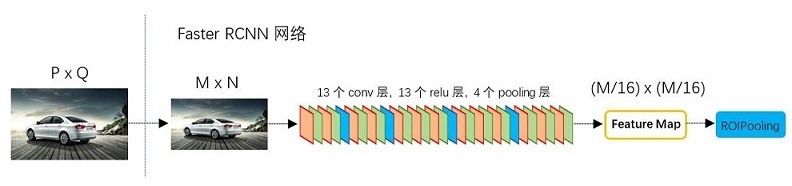Proposal Layer forward 按照以下顺序依次处理：

1. 再次生成anchors，并对所有的anchors做bbox reg位置回归（注意这里的anchors生成顺序和之前是即完全一致的）
2. 按照输入的foreground softmax scores由大到小排序anchors，提取前pre_nms_topN(e.g. 6000)个anchors。即提取修正位置后的foreground anchors
3. 利用feat_stride和im_info将anchors映射回原图，判断fg anchors是否大范围超过边界，剔除严重超出边界fg anchors。
4. 进行nms（nonmaximum suppression，非极大值抑制
5. 再次按照nms后的foreground softmax scores由大到小排序fg anchors，提取前post_nms_topN(e.g. 300)结果作为proposal输出。

RPN网络结构就介绍到这里，总结起来就是：

1. 生成 anchors
2. softmax 分类器提取 fg anchors
3. bbox reg 回归 fg anchors
4. Proposal Layer 生成 proposals

## 4 RoI pooling

1. 从图像中crop一部分传入网络
2. 将图像warp成需要的大小后传入网络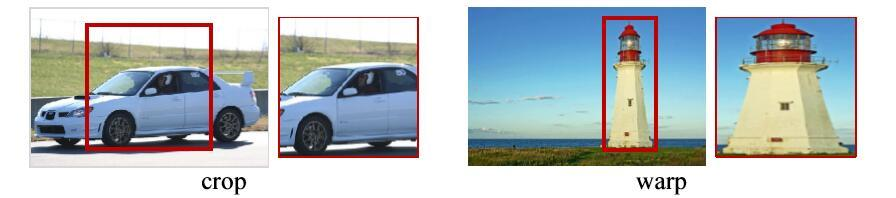ROI Pooling的过程就是将一个个大小不同的box矩形框，都映射成大小为$$w\times h$$的矩形框；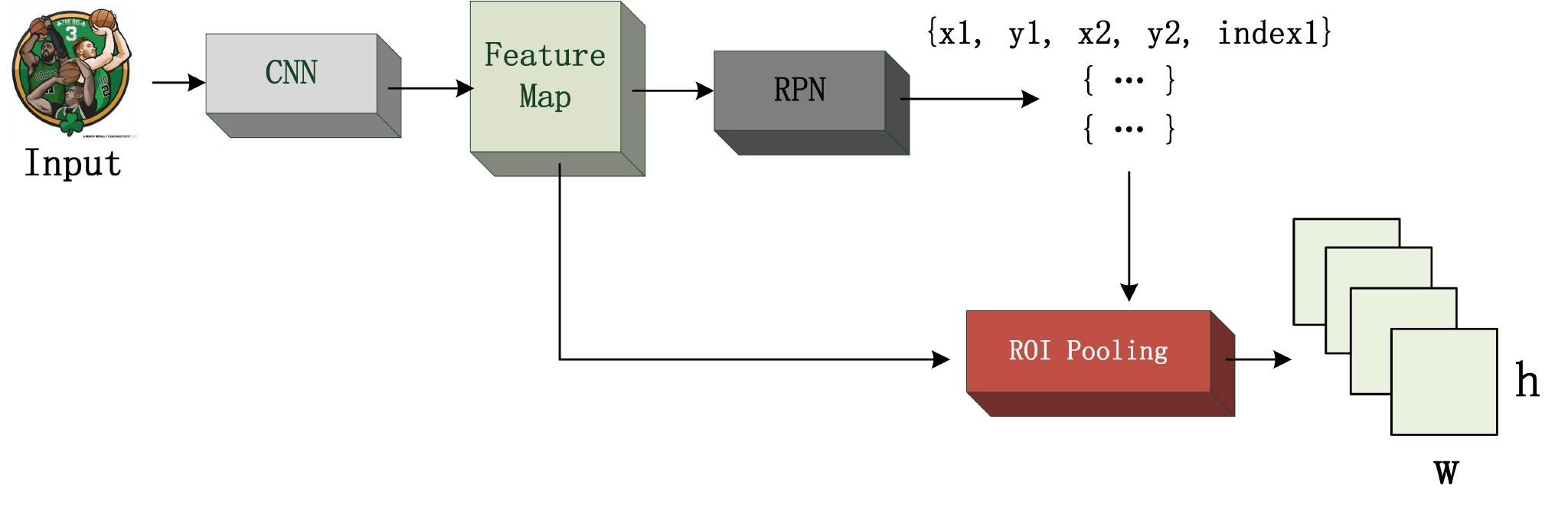RoI Pooling层则负责收集proposal，并计算出proposal feature maps，送入后续网络。Rol pooling层有2个输入：

1. 原始的feature maps（指的是进入RPN层之前的那个Conv层的Feature Map，通常我们称之为 share_conv）
2. RPN输出的proposal boxes（大小各不相同），其中值得注意的是：坐标的参考系不是针对feature map这张图的，而是针对原图的（神经网络最开始的输入）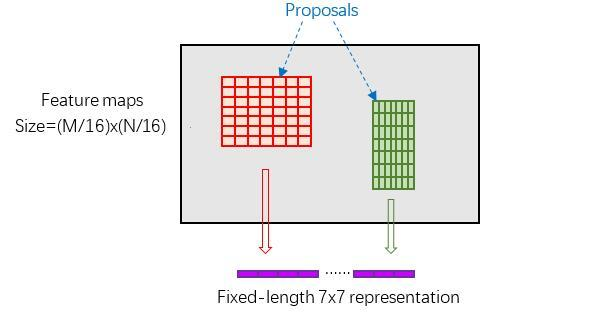## 5 Classification

Classification部分利用已经获得的proposal feature maps，通过full connect层与softmax计算每个proposal具体属于那个类别（如人，车，电视等），输出cls_prob概率向量；同时再次利用bounding box regression获得每个proposal的位置偏移量bbox_pred，用于回归更加精确的目标检测框。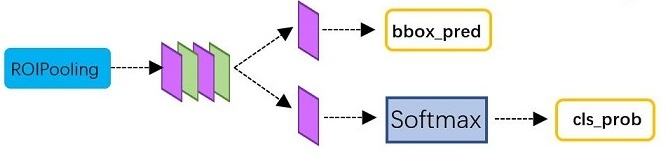## 6 Faster RCNN 训练

Faster CNN的训练，是在已经训练好的model（如VGG_CNN_M_1024，VGG，ZF）的基础上继续进行训练。实际中训练过程分为6个步骤：

1. 在已经训练好的model上，训练RPN网络
2. 利用步骤1中训练好的RPN网络，收集proposals
3. 第一次训练Fast RCNN网络
4. 第二训练RPN网络
5. 再次利用步骤4中训练好的RPN网络，收集proposals
6. 第二次训练Fast RCNN网络

RPN通过反向传播（BP，back-propagation）和随机梯度下降（SGD，stochastic gradient descent）进行端到端（end-to-end）训练。

$$L({p_{i}},{t_{i}})={\frac {1} {N_{cls}}}\sum_{i} L_{cls}(p_{i},p_{i}^{*}) + \lambda {\frac {1}{N_{reg}}} \sum_{i}p_{i}^{*} L_{reg}(t_i,t_i^{*})$$

$$p_i$$ 为 anchor 预测为目标的概率（foreground softmax predict概率）；

$$p_i^{*}$$代表对应的GT predict概率：

• 即当第i个anchor与GT间 $$IoU>0.7$$，认为是该anchor是foreground，$$p_i^{*}=1$$；
• 反之 $$IoU<0.3$$ 时，认为是该anchor是background，$$p_i^{*}=0$$；
• 至于那些 $$0.3<IoU<0.7$$ 的anchor则不参与训练。

$$p_i^*=\begin{cases} 0\ \ negative\ label\\ 1\ \ positive\ label\ \end{cases}$$

$$L_{cls}(p_i,p_i^*)$$ 是两个类别（目标 vs. 非目标）的对数损失：

$$L_{cls}(p_i,p_i^{*})=-\log [p_{i}^{*}p_i+(1-p_i^{*})(1-p_i)]$$

$$t_i=\{t_x,t_y,t_w,t_h\}$$ 是一个向量，表示预测的 bounding box 包围盒的4个参数化坐标；

$$t_i^*$$ 是与 positive anchor 对应的 ground truth （GT box）包围盒的坐标向量；

$$L_{reg}(t_i,t_i^*)$$ 是回归损失，用 $$L_{reg}(t_i,t_i^*)=R(t_i-t_i^*)$$ 来计算，$$R$$ 是 $$smooth L1$$ 函数：

$$L_{reg}(t_i,t_i^*)={\sum_{i \in {x,y,w,h}} smooth_{L1}(t_i-t_i^*)}$$

$$smooth_{L_{1}}(x) = \begin{cases} 0.5x^2, & \text{if |x| < 1}\\ |x|-0.5, & \text{otherwise} \end{cases}$$

smooth L1 函数图像为：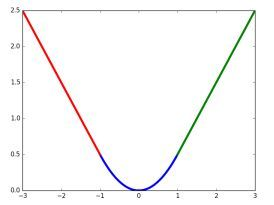1. cls loss，即rpn_cls_loss层计算的softmax loss，用于分类anchors为forground与background的网络训练
2. reg loss，即rpn_loss_bbox层计算的soomth L1 loss，用于bounding box regression网络训练。